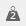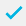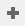# Calculating marks in a quiz, test or assessment

Using a weight matrix gives the ability to differentiate between correct or incorrect responses to a question.

In this example, four multiple choice questions are used, each with four possible answers. Using weighted variables we identify the response for a correct answer as those weighted as one and for an incorrect answer as those weighted as zero. Using these numbers the total number of correct responses can be calculated.

## Insert the questions

1. Create a multiple choice question with a single response.
1. Select Q1 and click Cloneto set up three more multiple choice questions called Q2, Q3 and Q4, each with four possible answers (codes) and with a single response.

## Create the weights

1. Click on the weightsbutton to open the Weights window.
2. Select New Weightto create a new weight. In Name enter ScoreA. This will be used for each question where A is the correct answer.
In Code List, enter a Value of 1 for Code 1 and 0 for Code 2, 3 and 4.
1. Click Saveto save the new weight and close the Weight Details window.
2. Repeat steps 2 to 3 to create three more similar weights for ScoreB, ScoreC and ScoreD where the value of 1 is in codes 2, 3 and 4 respectively.

## Create the derived variable

1. Open the Variables windowand clickto add a new variable which will be used to total the number of correct responses.
2. In Name enter Total.
3. Set the Type to Derived and the Response to Quantity.
4. In the Code list set the value for OK as ScoreA(Q1)+ScoreB(Q2)+ScoreC(Q3)+ScoreD(Q4)

The OK value is used as the total score. This depends on the correct answers for each question. In the example, Q1 has the answer A (the first answer), Q2 has the answer B (the second answer), Q3 has the answer C (the third answer) and Q4 has the answer D (the fourth answer).

## Display the result

You can display the total score to the participant.

1. Place the cursor in the text area of a question, title or instruction where you want the total to appear.
2. Right click on the cursor and click Insert and then click Variable Field.
3. In the New variable field window select Total in the Variable list and click OK.

The variable field appears like this in your questionnaire.

When your survey is completed it will appear like this in the browser.

If there is a topic you would like a worksheet on, email to snapideas@snapsurveys.com

Contents